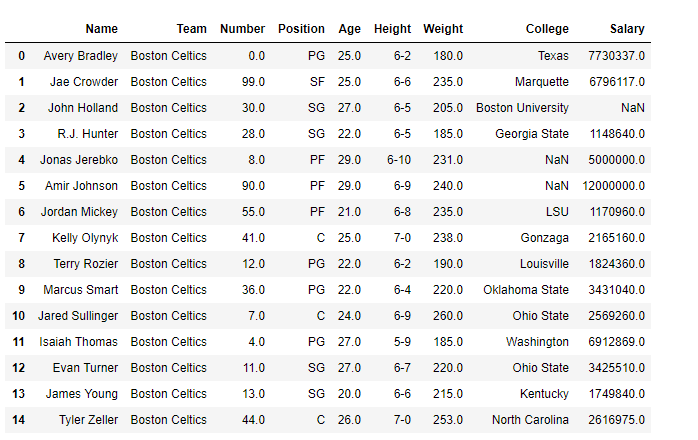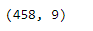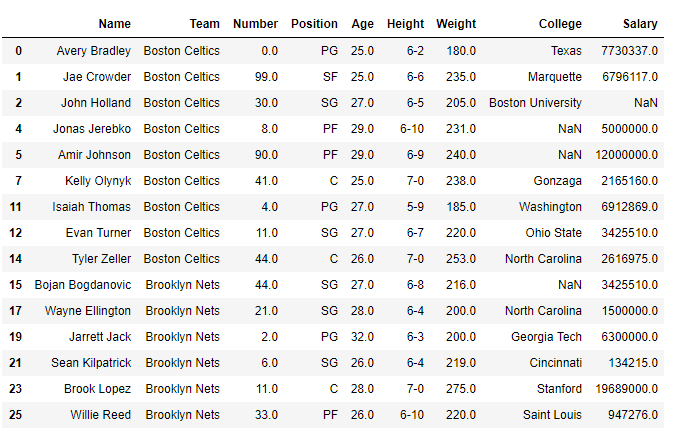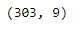# Drop rows from the dataframe based on certain condition applied on a column

Pandas provides a rich collection of functions to perform data analysis in Python. While performing data analysis, quite often we require to filter the data to remove unnecessary rows or columns.

We have already discussed earlier how to drop rows or columns based on their labels. However, in this post we are going to discuss several approaches on how to drop rows from the dataframe based on certain condition applied on a column. Retain all those rows for which the applied condition on the given column evaluates to `True`.

You are given the “nba.csv” dataset. Drop all the players from the dataset whose age is below 25 years.

Solution #1 : We will use vectorization to filter out such rows from the dataset which satisfy the applied condition.

 `# importing pandas as pd ` `import` `pandas as pd ` ` `  `# Read the csv file and construct the  ` `# dataframe ` `df ``=` `pd.read_csv(``'nba.csv'``) ` ` `  `# Visualize the dataframe ` `print``(df.head(``15``) ` ` `  `# Print the shape of the dataframe ` `print``(df.shape) `

Output :In this dataframe, currently, we are having 458 rows and 9 columns. Let’s use vectorization operation to filter out all those rows which satisfy the given condition.

 `# Filter all rows for which the player's ` `# age is greater than or equal to 25 ` `df_filtered ``=` `df[df[``'Age'``] >``=` `25``] ` ` `  `# Print the new dataframe ` `print``(df_filtered.head(``15``) ` ` `  `# Print the shape of the dataframe ` `print``(df_filtered.shape) `

Output :As we can see in the output, the returned dataframe only contains those players whose age is greater than or equal to 25 years.

Solution #2 : We can use the `DataFrame.drop()` function to drop such rows which does not satisfy the given condition.

 `# importing pandas as pd ` `import` `pandas as pd ` ` `  `# Read the csv file and construct the  ` `# dataframe ` `df ``=` `pd.read_csv(``'nba.csv'``) ` ` `  `# First filter out those rows which ` `# does not contain any data ` `df ``=` `df.dropna(how ``=` `'all'``) ` ` `  `# Filter all rows for which the player's ` `# age is greater than or equal to 25 ` `df.drop(df[df[``'Age'``] < ``25``].index, inplace ``=` `True``) ` ` `  `# Print the modified dataframe ` `print``(df.head(``15``)) ` ` `  `# Print the shape of the dataframe ` `print``(df.shape) `

Output :As we can see in the output, we have successfully dropped all those rows which do not satisfy the given condition applied to the ‘Age’ column.

My Personal Notes arrow_drop_upCheck out this Author's contributed articles.

If you like GeeksforGeeks and would like to contribute, you can also write an article using contribute.geeksforgeeks.org or mail your article to contribute@geeksforgeeks.org. See your article appearing on the GeeksforGeeks main page and help other Geeks.

Please Improve this article if you find anything incorrect by clicking on the "Improve Article" button below.

Article Tags :

1

Please write to us at contribute@geeksforgeeks.org to report any issue with the above content.